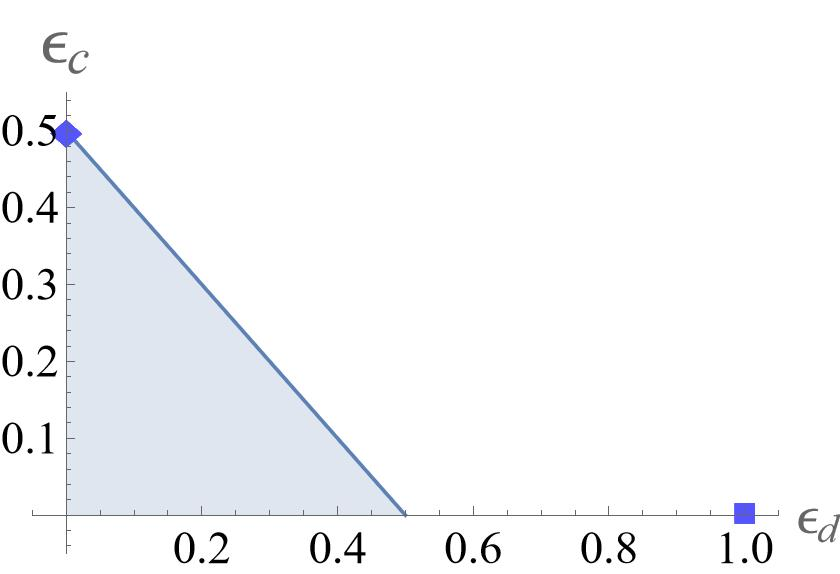# Privacy and correctness trade-offs for information-theoretically secure quantum homomorphic encryption

Homomorphic encryption, which allows a server to compute on encrypted data of a client without first decrypting it, is like a “Swiss army knife” of classical cryptography. Namely, by treating homomorphic encryption as a cryptographic primitive, a broad range of cryptographic notions such as multiparty secure computation and private information retrieval can be constructed. These reductions rely crucially on the data and circuit privacy of homomorphic encryption schemes. While data privacy is inherent in homomorphic encryption, circuit privacy encapsulates the property that no information about the computation circuit is leaked beyond its action on the encoded data. In this work, we make progress in understanding the extent in which quantum homomorphic encryption can be analogously a “Swiss army knife” of quantum cryptography. In particular, we explore the limitations of quantum homomorphic encryption in the paradigm of information-theoretic security.

Quantum homomorphic encryption that allows the delegation of an arbitrary quantum computation while also assuring the privacy of client’s encrypted data cannot exist, because its existence would violate well known information-theoretic bounds, such as Nielsen’s no-programming bound or fundamental coding-type bounds. On the other hand, if we consider quantum homomorphic encryption schemes that support only Clifford computations, such schemes exist with asymptotically perfect data-privacy and correctness. Hence, there is the hope that, by restricting ourselves to quantum homomorphic encryption schemes that support a limited set of operations, such schemes can still have enough functionality (data privacy, correctness and circuit privacy) to serve as a Swiss army knife. Here we show that this is not possible. Namely, even if we restrict ourselves to quantum homomorphic encryption protocols that can perform only two-qubit Clifford gates, we find that data privacy, circuit privacy and correctness cannot be simultaneously achieved. In particular, we obtain non-trivial trade-offs between these parameters for such computationally-restricted quantum homomorphic encryption schemes.

Our main contributions are as follows. First, we introduce a formal definition of information-theoretically secure circuit privacy for quantum homomorphic encryption schemes, which make use of a simulation scheme. Second, we give an explicit reduction from quantum oblivious transfer to quantum homomorphic encryption. In this reduction, we use only quantum homomorphic encryption protocols that perform delegated Clifford circuits, and which additionally utilize genuine random classical bits. Third, the reduction then allows us to inherit no-go results for quantum oblivious transfer to quantum homomorphic encryption. We find that, for any information-theoretically secure quantum homomorphic encryption scheme support (at least) Clifford operations, data privacy, circuit privacy and correctness cannot be simultaneously satisfied, and there is a trade-off between them.

As a concrete example, we illustrate our trade-off bound for perfect correctness. The blue line is the trade-off bound, and the shaded area is the forbidden area by the trade-off bound. The diamond corresponds to the trivial quantum homomorphic encryption protocol, and the square corresponds to the permutation quantum homomorphic encryption protocol.Our work has been presented on QCrypt 2022. Interested readers may find the full version in Arxiv.

This entry was posted in Uncategorized. Bookmark the permalink.